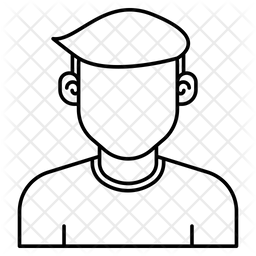# Can I estimate the detectable differences with Web Power?

936 viewsHello, Im trying to perform a post-hoc analysis to estimate the detectable differences (percentage difference of detectable

treatment means relative to control means) for a = 0.05 and a power of 80%. Does Web Power do this?...I have been

trying but  cant find the correct way. Want to estimate the detectable differences of a t-test (two tailed).

If anyone can help me it would be great!!

My data are:

sample 1) N= 16; mean= 122.4; S.E ± 8.38
Sample 2) N = 20; mean= 111.4; S.E. ± 6.35

Matías

+1 voteby johnny (3.3k points)

In the interface, if you leave the effect size field blank but supply the sample size, it will output the effect size to achieve the desired power. The effect size is also called the detectable difference or minimum detectalbe difference.asked Mar 10, 2016 by garma55 (180 points) but in that case, where do I put the mean values of my samples?by johnny (3.3k points)

There is no need to input mean value and standard deviation. The purpose is to estimate the effect size which is a function of mean and standard deviation. You would need to input information on sample size and power. The output is the effect size. Note that the sample effect size is equal to the ratio of mean or mean different divided by the standard devaition, you can calculate the mean or mean difference to be the effect size multiplied by the standard deviation. But usually, effect size itself is enough.

+1 voteby johnny (3.3k points)
edited by johnny

Let me provide a little more details here.

First, use the two-sample t-test power analysis, one can get the effect size is 0.967 with a power of 0.8 and the sample sizes $$n_1=16$$ and $$n_2 = 20$$.

Suppose the the common standard deviation of the two population can be estimated using the pooled standard deviation such that

$s_p = \sqrt{ \frac{(n_1-1)s_1^2+(n_2-1)s_2^2}{n_1+n_2-2} } = 7.32$

assuming $$s_1=8.38$$ and $$s_2=6.35$$. Then the detected difference should be 0.967*7.32 = 7.07.asked Mar 14, 2016 by garma55 (180 points) But why you equalize S with the standard error (S.E.)?